Get inspired by the success stories of our students in IIT JAM MS, ISI  MStat, CMI MSc DS.  Learn More

# ISI MStat PSB 2005 Problem 5 | Uniformity of UniformThis is a simple and elegant sample problem from ISI MStat PSB 2005 Problem 5. It's based the mixture of Discrete and Continuous Uniform Distribution, the simplicity in the problem actually fools us, and we miss subtle happenings. Be careful while thinking !

## Problem- ISI MStat PSB 2005 Problem 5

Suppose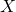and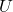are independent random variables with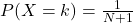,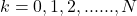,

andhaving a uniform distribution on [0,1]. Let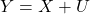.

(a) For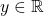, find.

(b) Find the correlation coefficient betweenand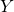.

### Prerequisites

Uniform Distribution

Law of Total Probability

Conditional Distribution

## Solution :

This ptroblem is quite straight forward enough, and we do what we are told to.

Here, we need to find the Cdf of, where ,, andandare defined as above.

So,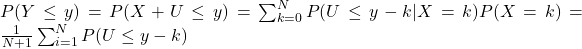, [ sinceandare indepemdent ],

Now, here is where we get fooled often by the simplicity of the problem. The beauty is to observe in the above expression, ifthen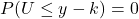, and ifthen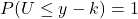, ( why??)

So, for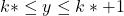the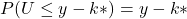, so when,for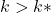, andfor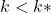.

So, the required Cdf will depend on the interval , y belongs to, for the above illustrated case, i.e.there will be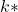number of 1's, and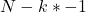number of 0's in the above summation, derived, so,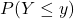reduces to,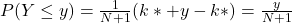,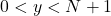, [ since heremay vary from 0,1,...., N, hence union of all the nested sub-intervals give the mentioned interval]

Hence the required Cdf. But can you find this Cdf argumentatively, without this algebraic labor ?? What distribution is it ?? Does the Uniform retains its Uniformity ?

I leave the part (b) as an exercise, its quite trivial.

## Food For Thought

How to deal with some random triangles ??

Suppose, You are given a stick of length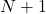units, Now you are to break the stick into 3 pieces, and the breaking points are chosen randomly , What is the chance of constructing a triangle with those 3 broken part as the sides of the constructed triangle ??

Now if you first break the stick into two pieces randomly, and further break the longer piece again into two parts (randomly), How the chance of making a triangle changes ?? Do the chance increases what do you think ??

Lastly, does the length of the stick matters at all , Give it a thought !!

## Subscribe to Cheenta at Youtube

This is a simple and elegant sample problem from ISI MStat PSB 2005 Problem 5. It's based the mixture of Discrete and Continuous Uniform Distribution, the simplicity in the problem actually fools us, and we miss subtle happenings. Be careful while thinking !

## Problem- ISI MStat PSB 2005 Problem 5

Supposeandare independent random variables with,,

andhaving a uniform distribution on [0,1]. Let.

(a) For, find.

(b) Find the correlation coefficient betweenand.

### Prerequisites

Uniform Distribution

Law of Total Probability

Conditional Distribution

## Solution :

This ptroblem is quite straight forward enough, and we do what we are told to.

Here, we need to find the Cdf of, where ,, andandare defined as above.

So,, [ sinceandare indepemdent ],

Now, here is where we get fooled often by the simplicity of the problem. The beauty is to observe in the above expression, ifthen, and ifthen, ( why??)

So, forthe, so when,for, andfor.

So, the required Cdf will depend on the interval , y belongs to, for the above illustrated case, i.e.there will benumber of 1's, andnumber of 0's in the above summation, derived, so,reduces to,,, [ since heremay vary from 0,1,...., N, hence union of all the nested sub-intervals give the mentioned interval]

Hence the required Cdf. But can you find this Cdf argumentatively, without this algebraic labor ?? What distribution is it ?? Does the Uniform retains its Uniformity ?

I leave the part (b) as an exercise, its quite trivial.

## Food For Thought

How to deal with some random triangles ??

Suppose, You are given a stick of lengthunits, Now you are to break the stick into 3 pieces, and the breaking points are chosen randomly , What is the chance of constructing a triangle with those 3 broken part as the sides of the constructed triangle ??

Now if you first break the stick into two pieces randomly, and further break the longer piece again into two parts (randomly), How the chance of making a triangle changes ?? Do the chance increases what do you think ??

Lastly, does the length of the stick matters at all , Give it a thought !!

## Subscribe to Cheenta at Youtube

This site uses Akismet to reduce spam. Learn how your comment data is processed.

### Knowledge Partner